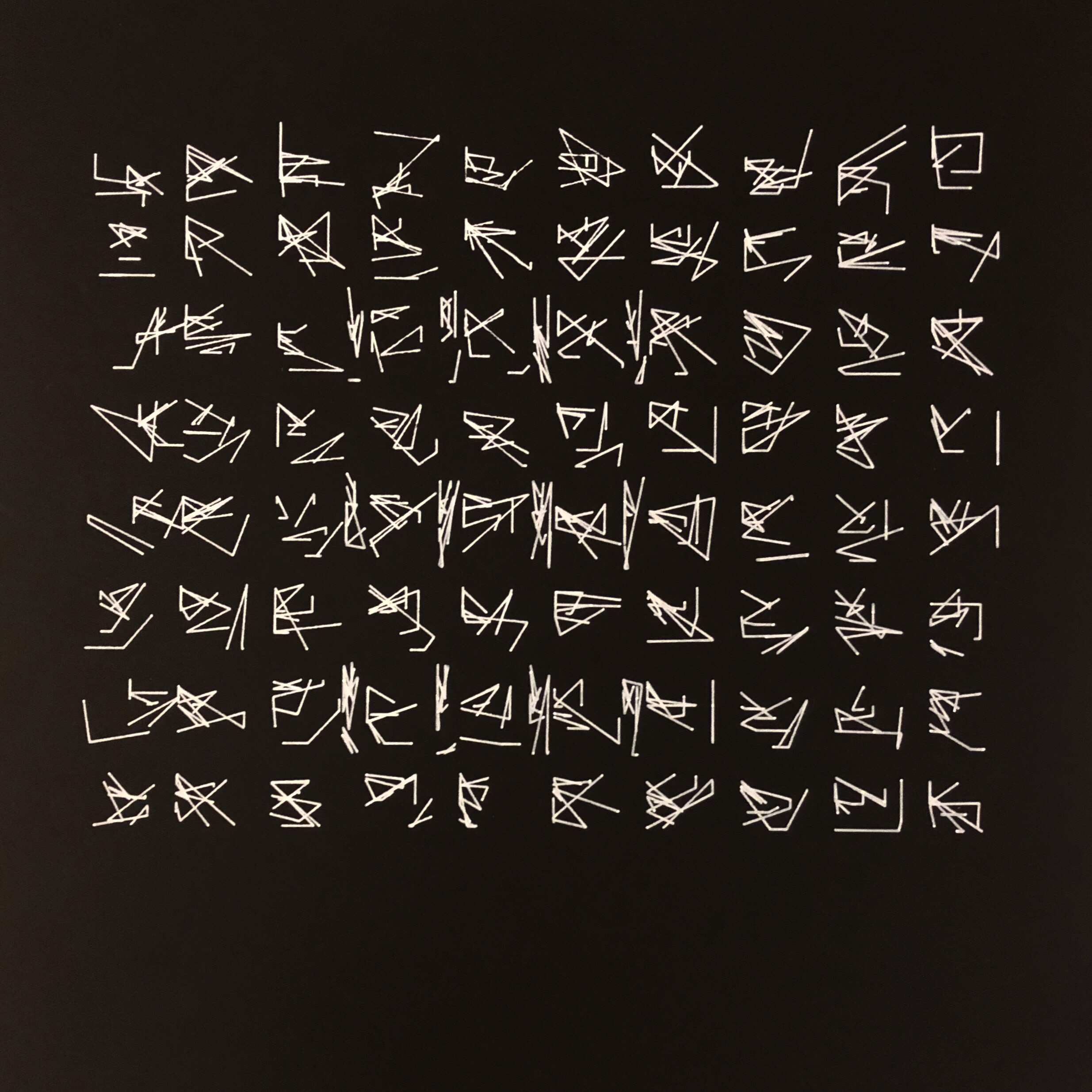# Ackso-Asemic

Since asemic writing gives the illusion of meaning, I wanted to use simple, abstract elements to give the illusion of complexity. I generated random points on the perimeter of squares, connected them, and then focussed on a random portion of each character and repeated the process. I’ve found that recursive methods like this are optimal for creating visual/compositional complexity since fractal-like forms tend to draw the eye to the most detailed part of an image without wasting details. Conveniently, this results in most of the lines being connected, but there are often small separated fragments, which looks deliberate and punctual. In the third to sixth margins, I added some long, thin characters to break up the grid. These also function as annotations, and add to the composition in the same way as the principles that defined my characters–by taking a random portion of the frame and adding smaller details without clearly separating them from what was already there.

plot```add_library('pdf')   bRecordingPDF = False pdfOutputCount = 0   def setup(): size(1000, 1000) global bRecordingPDF bRecordingPDF = True #noLoop()   def drawWord(x,y,w,h, points,curses):   if (curses&lt;1): pass else: pointlist= [] for i in range(points): rand = int(random(0,3)) if rand==0: pointlist.append([x,random(y,h+y)]) elif rand==1: pointlist.append([w+x,random(y,h+y)]) elif rand==2: pointlist.append([random(x,x+w),y+h]) else: pointlist.append([random(x,x+w),y])   for i in range(len(pointlist)-1): line(pointlist[i],pointlist[i],pointlist[i+1],pointlist[i+1]) factor=.75 w=factor*w h=factor*h x+=(random(0,(w-x))) y+=(random(0,(h-y))) return drawWord(x,y,w,h,points,curses-1)     def keyPressed(): bRecordingPDF = True   def mousePressed(): background(255) grid(0,0,500,500,10,35,35) grid(137,90,200,400,4,10,50)     def grid(x,y,w,h,cols,s,q): side=w/cols up=h/cols for i in range(cols): for p in range(cols): drawWord(i*side+x,p*up+y,s,q,4,4)     def draw():     global bRecordingPDF global pdfOutputCount if (bRecordingPDF): background(255) beginRecord(PDF, "ackso" + str(pdfOutputCount) + ".pdf") background(255) grid(0,0,500,500,10,35,35) grid(137,90,200,400,4,10,50)     endRecord() bRecordingPDF = False pdfOutputCount+=1```